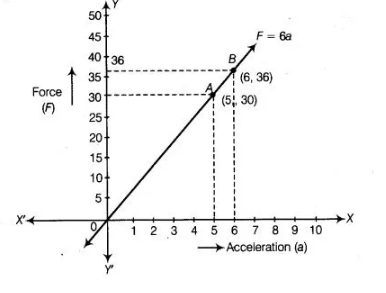# The force exerted to pull a cart is directly`
Question:

The force exerted to pull a cart is directly proportional to the acceleration produced in the body. Express the statement as a linear equation of two

variables and draw the graph of the same by taking the constant mass equal to 6 kg. Read from the graph, the force required when the acceleration

produced is

(i) 5 ms-2

(ii) 6 ms-2

Thinking Process

(i) Firstly, make a proportionality equation in terms of force (F) and acceleration (a) i.e., F°∝ a.

(ii) Secondly, write the proportional equation in linear equation by using arbitrary constant 6.

(iii) Further, for two different values of a we get the values of F and plot these points on a graph paper and join them to get the line.

Solution:

Given that, the force $(F)$ is directly proportional to the acceleration $(a)$.

i.e., $\quad F \propto \mathrm{a}$

$\Rightarrow \quad F=m a$ [where, $m=$ arbitrary constant and take value $6 \mathrm{~kg}$ of mass]

$\therefore$ $F=6 a$ $\ldots(i)$

(i) If $a=5 \mathrm{~m} / \mathrm{s}^{2}$, then from Eq. (i), we get

$F=6 \times 5=30 \mathrm{~N}$

(ii) If $a=6 \mathrm{~m} / \mathrm{s}^{2}$, then from Eq. (i), we get

$F=6 \times 6=36 \mathrm{~N}$

Here, we find two points $A(5,30)$ and $B(6,36)$. So, draw the graph by plotting the points and joining the line $A B$.